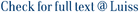We study the probabilistic properties of the fractional Ornstein–Uhlenbeck process, which is a relevant framework for volatility modeling in continuous time. First, we compute an expression for its variance for any value of the Hurst parameter, H ∈ (0, 1). Second, we derive the density of the process and we calculate the probability of its supremum to be above a given threshold. We provide a number of illustrations based on fractional stochastic volatility models, such as those of Comte and Renault (1998), Bayer et al. (2016) and Gatheral et al. (2018). Finally, the empirical analysis, based on the realized variance series of S&amp;P500, shows the usefulness of these theoretical results for risk management purposes, especially when a characterization of the volatility tail risk is needed.

Volatility tail risk under fractionality / Morelli, Giacomo; Santucci de Magistris, Paolo. - In: JOURNAL OF BANKING & FINANCE. - ISSN 1872-6372. - 108:(2019), pp. 1-9. [10.1016/j.jbankfin.2019.105654]

### Volatility tail risk under fractionality

#### Abstract

We study the probabilistic properties of the fractional Ornstein–Uhlenbeck process, which is a relevant framework for volatility modeling in continuous time. First, we compute an expression for its variance for any value of the Hurst parameter, H ∈ (0, 1). Second, we derive the density of the process and we calculate the probability of its supremum to be above a given threshold. We provide a number of illustrations based on fractional stochastic volatility models, such as those of Comte and Renault (1998), Bayer et al. (2016) and Gatheral et al. (2018). Finally, the empirical analysis, based on the realized variance series of S&P500, shows the usefulness of these theoretical results for risk management purposes, especially when a characterization of the volatility tail risk is needed.
##### Scheda breve Scheda completa Scheda completa (DC)Fractional Ornstein–Uhlenbeck, Supremum, Rough volatility, VIX, VolaR
File in questo prodotto:
File
JBF2019b.pdf

Solo gestori archivio

Tipologia: Versione dell'editore
Licenza: DRM non definito
Dimensione 1.57 MB
Utilizza questo identificativo per citare o creare un link a questo documento: `http://hdl.handle.net/11385/189452`
•2
•1